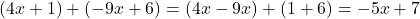Find an expression which represents the sum of (4x + 1) and (-9x + 6) in simplest terms.

Question

Find an expression which represents the sum of (4x + 1) and (-9x + 6) in

simplest terms.

in progress 0
6 months 2021-08-10T01:46:50+00:00 1 Answers 8 views 0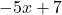Step-by-step explanation:

Given: Two expressions are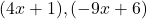To find: sum of the given expressions in simplest terms

Solution:

A variable is a term that takes various numerical values and a constant is a term that takes a fixed numerical value.

Consider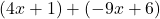Here, solve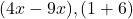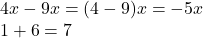Therefore,